# constructDefaultFixedEffectValues

Create structure containing initial estimates fixed effects needed for fit

## Syntax

``FEInitEstimates = constructDefaultFixedEffectValues(CovModel)``

## Description

example

````FEInitEstimates = constructDefaultFixedEffectValues(CovModel)` returns `FEInitEstimates`, a structure containing the initial estimates for the fixed effects in `CovModel`, a `CovariateModel` object. By default, the values of initial estimates are set to zero.```

## Examples

collapse all

Create an empty `CovariateModel` object.

`covModel = CovariateModel;`

Set its Expression property to define the relationships between parameters (Cl, V, and k) and covariate (w). You must use `theta` as a prefix for all fixed effects and `eta` for random effects.

`covModel.Expression = ["Cl = theta1 + theta2*w + eta1","V = theta3 + eta2","k = theta4 + eta3"];`

Display the names of fixed effects.

`covModel.FixedEffectNames`
```ans = 4x1 cell {'theta1'} {'theta3'} {'theta4'} {'theta2'} ```

The `FixedEffectDescription` property displays which fixed effects correspond to which parameter. For instance, theta1 is the fixed effect for the Cl parameter, and theta2 is the fixed effect for the weight covariate that has a correlation with Cl parameter, denoted as Cl/w.

`covModel.FixedEffectDescription`
```ans = 4x1 cell {'Cl' } {'V' } {'k' } {'Cl/w'} ```

Specify initial estimates for the fixed effects. Create a default structure containing initial estimates using the `constructDefaultFixedEffectValues` function.

`initialEstimates = constructDefaultFixedEffectValues(covModel)`
```initialEstimates = struct with fields: theta1: 0 theta3: 0 theta4: 0 theta2: 0 ```

Update the initial estimate value of each fixed effects.

```initialEstimates.theta1 = 1.20; initialEstimates.theta2 = 0.30; initialEstimates.theta3 = 0.90; initialEstimates.theta4 = 0.10;```

Update the `FixedEffectValues` property to use the updated initial estimates.

`covModel.FixedEffectValues = initialEstimates;`

Check the covariate model for errors.

`verify(covModel)`

Estimate nonlinear mixed-effects parameters using clinical pharmacokinetic data collected from 59 infants. Evaluate the fitted model given new data or dosing information.

This example uses data collected on 59 preterm infants given phenobarbital during the first 16 days after birth . `ds` is a table containing the concentration-time profile data and covariate information for each infant (or group).

`load pheno.mat ds`

Convert to groupedData

Convert the data to the groupedData format for parameter estimation.

`data = groupedData(ds);`

Display the first few rows of `data`.

`data(1:5,:)`
```ans = 5x6 groupedData ID TIME DOSE WEIGHT APGAR CONC __ ____ ____ ______ _____ ____ 1 0 25 1.4 7 NaN 1 2 NaN 1.4 7 17.3 1 12.5 3.5 1.4 7 NaN 1 24.5 3.5 1.4 7 NaN 1 37 3.5 1.4 7 NaN ```

Visualize Data

Display the data in a trellis plot.

```t = sbiotrellis(data, 'ID', 'TIME', 'CONC', 'marker', 'o',... 'markerfacecolor', [.7 .7 .7], 'markeredgecolor', 'r', ... 'linestyle', 'none'); t.plottitle = 'Concentration versus Time';```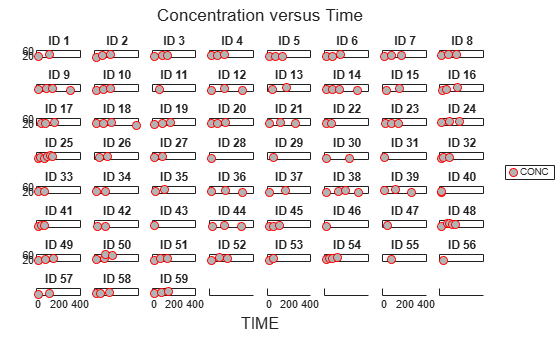Create a One-Compartment PK Model

Create a simple one-compartment PK model, with bolus dose administration and linear clearance elimination, to fit the data.

```pkmd = PKModelDesign; addCompartment(pkmd,'Central','DosingType','Bolus',... 'EliminationType','linear-clearance',... 'HasResponseVariable',true,'HasLag',false); onecomp = pkmd.construct;```

Map model species to response data.

`responseMap = 'Drug_Central = CONC';`

Define Estimated Parameters

The parameters to estimate in this model are the volume of the central compartment (`Central`) and the clearance rate (`Cl_Central`). `sbiofitmixed` calculates fixed and random effects for each parameter. The underlying algorithm computes normally distributed random effects, which might violate constraints for biological parameters that are always positive, such as volume and clearance. Therefore, specify a transform for the estimated parameters so that the transformed parameters follow a normal distribution. The resulting model is

`$log\left({V}_{i}\right)=log\left({\varphi }_{V,i}\right)={\theta }_{V}+{\eta }_{V,i}$`

and

`$log\left(C{l}_{i}\right)=log\left({\varphi }_{Cl,i}\right)={\theta }_{Cl}+{\eta }_{Cl,i},$`

where $\theta$, $eta$, and $\varphi$ are the fixed effects, random effects, and estimated parameter values respectively, calculated for each infant (group) $i$. Some arbitrary initial estimates for V (volume of central compartment) and Cl (clearance rate) are used here in the absence of better empirical data.

`estimatedParams = estimatedInfo({'log(Central)','log(Cl_Central)'},'InitialValue',[1 1]);`

Define Dosing

All infants were given the drug, represented by the `Drug_Central` species, where the dosing schedule varies among infants. The amount of drug is listed in the data variable DOSE. You can automatically generate dose objects from the data and use them during fitting. In this example, `Drug_Central` is the target species that receives the dose.

```sampleDose = sbiodose('sample','TargetName','Drug_Central'); doses = createDoses(data,'DOSE','',sampleDose);```

Fit the Model

Use `sbiofitmixed` to fit the one-compartment model to the data.

`nlmeResults = sbiofitmixed(onecomp,data,responseMap,estimatedParams,doses,'nlmefit');`

Visualize Results

Visualize the fitted results using individual-specific parameter estimates.

`plot(nlmeResults,'ParameterType','individual');`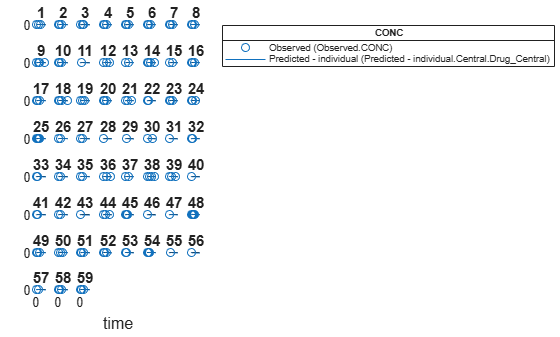Use New Dosing Data to Simulate the Fitted Model

Suppose you want to predict how infants 1 and 2 would have responded under different dosing amounts. You can predict their responses as follows.

Create new dose objects with new dose amounts.

```dose1 = doses(1); dose1.Amount = dose1.Amount*2; dose2 = doses(2); dose2.Amount = dose2.Amount*1.5;```

Use the `predict` function to evaluate the fitted model using the new dosing data. If you want response predictions at particular times, provide the new output time vector. Use the 'ParameterType' option to specify individual or population parameters to use. By default, `predict` uses the population parameters when you specify output times.

```timeVec = [0:25:400]; newResults = predict(nlmeResults,timeVec,[dose1;dose2],'ParameterType','population');```

Visualize the predicted responses while overlapping the experimental data for infants 1 and 2.

```figure; subplot(2,1,1) plot(data.TIME(data.ID == 1),data.CONC(data.ID == 1),'bo') hold on plot(newResults(1).Time,newResults(1).Data,'b') hold off ylabel('Concentration') legend('Observation(CONC)','Prediction') subplot(2,1,2) plot(data.TIME(data.ID == 2),data.CONC(data.ID == 2),'rx') hold on plot(newResults(2).Time,newResults(2).Data,'r') hold off legend('Observation(CONC)','Prediction') ylabel('Concentration') xlabel('Time')```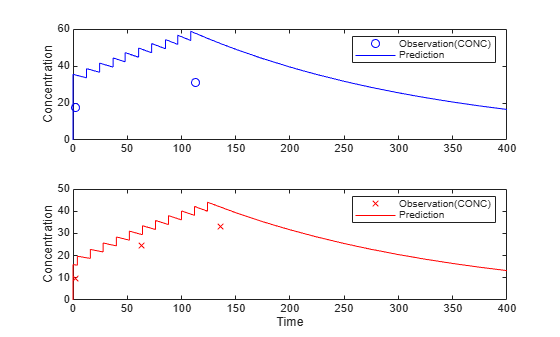Create a Covariate Model for the Covariate Dependencies

Suppose there is a correlation between volume and weight, and possibly volume and APGAR score. Consider the effect of weight by modeling two of these covariate dependencies: the volume of central (`Central`) and the clearance rate (`Cl_Central`) vary with weight. The model becomes

`$log\left({V}_{i}\right)=log\left({\varphi }_{V,i}\right)={\theta }_{V}+{\theta }_{V/weight}*weigh{t}_{i}+{\eta }_{V,i}$`

and

`$log\left(C{l}_{i}\right)=log\left({\varphi }_{Cl,i}\right)={\theta }_{Cl}+{\theta }_{Cl/weight}*weigh{t}_{i}+{\eta }_{Cl,i}$`

Use the `CovariateModel` object to define the covariate dependencies. For details, see Specify a Covariate Model.

```covModel = CovariateModel; covModel.Expression = ({'Central = exp(theta1 + theta2*WEIGHT + eta1)',... 'Cl_Central = exp(theta3 + theta4*WEIGHT + eta2)'});```

Use `constructDefaultInitialEstimate` to create an `initialEstimates` struct.

`initialEstimates = covModel.constructDefaultFixedEffectValues;`

Use the `FixedEffectNames` property to display the thetas (fixed effects) defined in the model.

`covModel.FixedEffectNames`
```ans = 4x1 cell {'theta1'} {'theta3'} {'theta2'} {'theta4'} ```

Use the `FixedEffectDescription` property to show the descriptions of corresponding fixed effects (thetas) used in the covariate expression. For example, `theta2` is the fixed effect for the weight covariate that correlates with the volume (`Central`), denoted as 'Central/WEIGHT'.

`disp('Fixed Effects Description:');`
```Fixed Effects Description: ```
`disp(covModel.FixedEffectDescription);`
``` {'Central' } {'Cl_Central' } {'Central/WEIGHT' } {'Cl_Central/WEIGHT'} ```

Set the initial guesses for the fixed-effect parameter values for `Central` and `Cl_Central` using the values estimated from fitting the base model.

```initialEstimates.theta1 = nlmeResults.FixedEffects.Estimate(1); initialEstimates.theta3 = nlmeResults.FixedEffects.Estimate(2); covModel.FixedEffectValues = initialEstimates;```

Fit the Model

`nlmeResults_cov = sbiofitmixed(onecomp,data,responseMap,covModel,doses,'nlmefit');`

Display Fitted Parameters and Covariances

`disp('Estimated Fixed Effects:');`
```Estimated Fixed Effects: ```
`disp(nlmeResults_cov.FixedEffects);`
``` Name Description Estimate StandardError __________ _____________________ ________ _____________ {'theta1'} {'Central' } -0.45664 0.078933 {'theta3'} {'Cl_Central' } -5.9519 0.1177 {'theta2'} {'Central/WEIGHT' } 0.52948 0.047342 {'theta4'} {'Cl_Central/WEIGHT'} 0.61954 0.071386 ```
`disp('Estimated Covariance Matrix:');`
```Estimated Covariance Matrix: ```
`disp(nlmeResults_cov.RandomEffectCovarianceMatrix);`
``` eta1 eta2 ________ ________ eta1 0.046503 0 eta2 0 0.041609 ```

Visualize Results

Visualize the fitted results using individual-specific parameter estimates.

`plot(nlmeResults_cov,'ParameterType','individual');`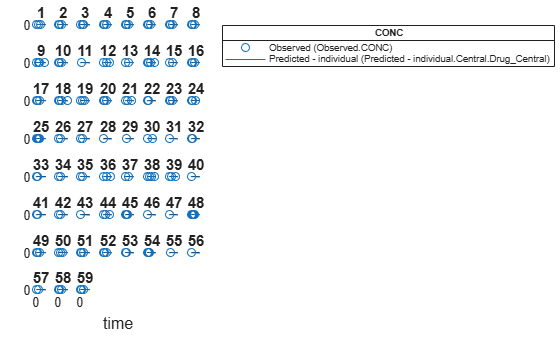Use New Covariate Data to Evaluate the Fitted Model

Suppose you want to explore the responses of infants 1 and 2 using different covariate data, namely `WEIGHT`. You can do this by specifying the new `WEIGHT` data. The `ID` variable of the data corresponds to individual infants.

```newData = data(data.ID == 1 | data.ID == 2,:); newData.WEIGHT(newData.ID == 1) = 1.3; newData.WEIGHT(newData.ID == 2) = 1.4;```

Simulate the responses of infants 1 and 2 using the new covariate data.

`[newResults_cov, newEstimates] = predict(nlmeResults_cov,newData,[dose1;dose2]);`

`newEstimates` contains the updated parameter estimates for each individual (infants 1 and 2) after the model is reevaluated using the new covariate data.

`newEstimates`
```newEstimates=4×3 table Group Name Estimate _____ ______________ _________ 1 {'Central' } 2.5596 1 {'Cl_Central'} 0.0065965 2 {'Central' } 1.7123 2 {'Cl_Central'} 0.0064806 ```

Compare to the estimated values from the original fit using the old covariate data.

```nlmeResults_cov.IndividualParameterEstimates( ... nlmeResults_cov.IndividualParameterEstimates.Group == '1' | ... nlmeResults_cov.IndividualParameterEstimates.Group == '2',:)```
```ans=4×3 table Group Name Estimate _____ ______________ _________ 1 {'Central' } 2.6988 1 {'Cl_Central'} 0.0070181 2 {'Central' } 1.8054 2 {'Cl_Central'} 0.0068948 ```

Visualize the new simulation results together with the experimental data for infant 1 and 2.

```figure; subplot(2,1,1); plot(data.TIME(data.ID == 1),data.CONC(data.ID == 1),'bo') hold on plot(newResults_cov(1).Time,newResults_cov(1).Data,'b') hold off ylabel('Concentration') legend('Observation(CONC)','Prediction','Location','NorthEastOutside') subplot(2,1,2) plot(data.TIME(data.ID == 2),data.CONC(data.ID == 2),'rx') hold on plot(newResults_cov(2).Time,newResults_cov(2).Data,'r') hold off legend('Observation(CONC)','Prediction','Location','NorthEastOutside') ylabel('Concentration') xlabel('Time')```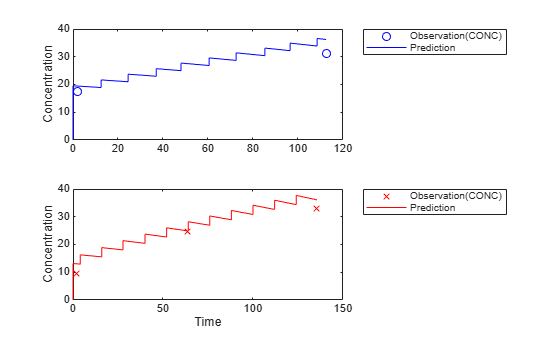References

 Grasela, T. H. Jr., and S. M. Donn. "Neonatal population pharmacokinetics of phenobarbital derived from routine clinical data." Dev Pharmacol Ther 1985:8(6). 374-83.

## Input Arguments

collapse all

Covariate model, specified as a `CovariateModel` object.

## Output Arguments

collapse all

Initial estimates for fixed effects, returned as a structure. Each field of the structure represents a fixed effect and its value. By default, the values of initial estimates are set to zero, but you can edit these estimates as needed. If you do, make sure that the number and names of the fields in the `FEInitEstimates` structure matches the number and names of fixed effects (`theta` values) in the Expression property of `CovModel`.

## Version History

Introduced in R2011b